• 回溯法求解八皇后问题
2018-03-22 17:30:05

回溯法求解八皇后问题，主要就是寻找状态空间树，然后利用判断函数剪去一些不符合的解，相比较枚举运算量更少

package com.huat.algorithm;

import java.util.Scanner;

/**
* @author yanzz
* @编辑时间:2018年3月22日
* @功能说明:N皇后，回溯法求解
*
* 	回溯法的即被概念:使用剪枝函数的深度优先方式生成的状态空间树中的结点求解方法称为回溯法
*
* 回溯法: 1)显示约束，确定每个xi取值的约束条件成为显示约束。
* 		2）隐式约束:给出判断一个候选解是否是可行解
* 		3）代价函数:用来衡量每个可行解的优劣，使目标函数去最值的可行解为问题的最优解
* 		4）状态空间树
* @version:
*/
public class NQueens {

//
private static boolean place(int k,int i, int[] tail) {
//判断两个皇后是否在同一列或在同一斜线上
for(int j = 0; j < k; j++) {

if(tail[j] == i || Math.abs(tail[j] - i) == Math.abs(j - k) ) {
return false;
}
}
return true;
}

public static void seek(int k, int n, int[] temp) {

for(int i = 0; i < n; i++) {		//显示约束

if(place(k,i,temp)) {			//约束函数
temp[k] = i;

if(k == n -1) {
for(int m : temp) {
System.out.printf("%d",m);
}
System.out.println();
} else {
seek(k+1,n,temp);
}
}
}
}

public static void main(String[] args) {
Scanner sc = new Scanner(System.in);
int n;
n = sc.nextInt();
int[] x = new int[n];	//最有解空间
seek(0,n,x);
sc.close();
}
}


更多相关内容
• 回溯法求解八皇后问题

问题提出：八皇后问题是一个古老而著名的问题。该问题是十九世纪著名的数学家高斯1850 提出在 8x8 格的国际象棋上摆放八皇后，使其不能互相攻击，即任意两个皇后都不能处于同一行、同一列或同一斜线上，问有多少种摆法。高斯认为有76种方案。1854年在柏林的象棋杂志上不同的作者发表了 40 种不同的解，后人有人用图论的方法解出 92种结果。虽然问题的关键在于如何判定某个皇后所在的行、列、斜线是否有别的皇后;可以从矩阵的特点上找到规律，如果在同一行，则行号相同:如果在同一列上，则列号相同;如果同在“/”斜线上的行列值之和相同:如果在对角线上，则行列号之和或行列号之差相等，逐个纪录符合题意的情况,最终得出解。

解决思路：利用数组来求解。

1)解决冲突问题:这个问题包括了行，列，两条对角线。

行:规定每一行放一个皇后，不会造成行上的冲突;

列:当第1列被某个皇后占领后，则同一列上的所有空格都不能再放皇后，被标记位置为被占领状态。

对角线：对角线有两个方向，在同一对角线上的所有点都不能有冲突。

2)使用算法分析与设计的知识，用递归法、枚举法、回溯法等解决问题。

3）思考要解决的问题：用什么算法分析与设计来描述棋盘，怎样描述棋盘，怎样描述皇后（包括皇后的位置，怎样移动皇后等）。怎样开始程序。

4）初步解决问题，列出大纲。

所用算法

回溯法：

回溯算法也叫试探法，它是一种系统地搜索问题的解的方法。按选优条件向

前搜索，以达到目标。当探索到某一步时，发现原先选择并不优或达不到目标， 就退回一步重新选择，这种走不通就退回再走的技术为回溯法，而满足回溯条件的某个状态的点称为“回溯点”。

行A的皇后放在第一列以后，行B的皇后放在第一列已经发生冲突。这时候不必继续放行C的皇后，而是调整行B的皇后到第二列，继续冲突放第三列，不冲突了才开始进入行C。如此可依次放下行A至E的皇后，将每个皇后往右边横向、斜向攻击的点位用叉标记，发现行F的皇后无处安身。这时回溯到行E的皇后，将其位置由第4列调整为第8列，进入行F，发现皇后依然无处安身，再次回溯行E。此时行E已经枚举完所有情况，回溯至行D，将其由第2列移至第7列，再进入行E继续。按此算法流程最终找到可行解，成功在棋盘里放下了8个“和平共处”的皇后。继续找完全部的解共92个。

回溯算法求解八皇后问题的原则是：有冲突解决冲突，没有冲突往前走，无路可走往回退，走到最后是答案。

### 流程图：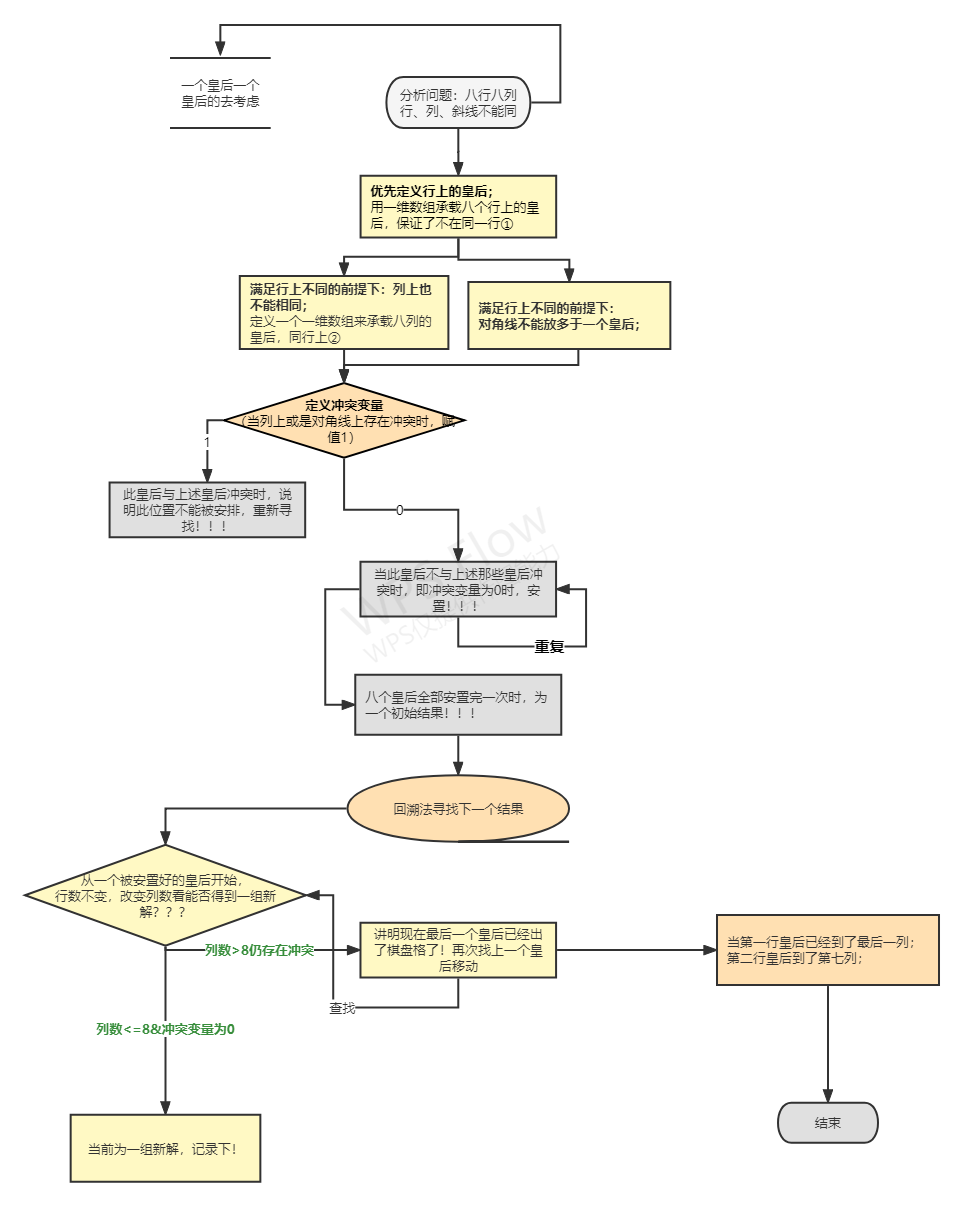运行代码：

#include <stdio.h>

int queen = {0};

int sum = 0;

int cnt ; //表示摆放了几个皇后，也表示摆放皇后的行数。

int col ; //表示在这一列上摆放了皇后

void greedy(){

while(1){

//在(cnt,col)这个坐标摆放皇后

if(cnt == 1 && queen == 7 && col == 6){ //表示第一行的皇后已经到了第八列且第二行的皇后到了第六列位置，已经摆放不下皇后了就退出循环

break;

}

int isAttack = 0; //用来表示皇后们之间是否能够攻击的到，如果攻击的到就是1，否则就为0

int i=0;

for(i=0;i<cnt;i++){

if(queen[i] == col){ //表示在同一列上

isAttack = 1;

}

int div_row =cnt -i; //表示斜线上的纵坐标之差

int div_col = queen[i]-col; //表示斜线上横坐标之差

if(div_row == div_col ||div_row == -div_col){ //表示在同一斜线上

isAttack = 1;

}

}

if(isAttack == 0){ //表示可以放置

queen[cnt] = col; //记录皇后当前的列数

cnt++; //开始摆放下一个皇后

col = 0; //下一个皇后从第一列开始遍历

if(cnt == 8){ //如果摆满了八个皇后就打印出他们的摆法

for (i = 0 ; i <= 7; ++i) //打印该种摆放方法情况

{

for (int col=0;col<=7;col++)

{

if (col!=queen[i])

{

printf("0|");

}

else{printf("1|");}

}

printf("\n");

}

for(i=0;i<8;i++){

printf("%d  ",queen[i]+1);

}

printf("\n");

sum++;

printf("这是第%d种摆法\n",sum);

printf("\n"); //并且摆放种数+1

do{ //越界问题 //回朔

cnt--; //撤回正在摆放的皇后

col = queen[cnt]+1; //往下一个列寻找摆放位置

}while(col>=8);

}

}else{ //表示不能摆放

col++;

while(col>=8){ //回朔

cnt--; //退一格

col = queen[cnt]+1; //上一个皇后往后移一格

}

}

}

printf("总共有%d种摆法\n",sum);

return ;

}

int main(){

greedy();

return 0;

}

### 总结感悟：

1.心得体会

这次课程设计我们解决了八皇后问题的位置输出问题，利用回溯法完成了八皇后九十二种解法。本问题的解题思路使用的是典型的回溯算法。回溯法有“通用解题法”之称。用它可以系统地搜索一个问题的所有解或任一解。回溯法在问题的解空间树中，拨深度优先策略，从根节点出发搜索解空间树。算法搜索至解空间树的任一结点时，先判断该结点是否包含问题的解。如果肯定不包含，则跳过对以该结点为根的子树的搜索，逐层向其祖先结点回溯。否则，进入该子树，继续按深度优先策略搜索。它适合于解组合数较大的问题。

2.总结

此次的八皇后课程设计编程过程中，我们从最开始的查资料、确定算法、翻找课件、寻求递归过程一系列流程中，我们小组三人都熟悉掌握了算法设计与分析课程中的回溯、递归的应用，协同完善了八皇后安置问题。通过团队合作，使我们更好的解决问题，每个人都负责不同模块的操作，大大提高了效率。另外网络资料、向他人寻求帮助那些自己掌握不是很深刻的知识点都得到了巩固，大大提高了效率，更是明白了不同算法带来的效果是大大不同的，通过分析推敲我们选择了回溯算法，这种思维更有助于我们解决其他的问题！

展开全文• 八皇后问题，是一个古老而著名的问题，是回溯算法的典型案例。该问题是国际西洋棋棋手马克斯·贝瑟尔于1848年提出：在8x8格的国际象棋上摆放八个皇后，使其不能互相攻击，即任意两个皇后都不能处于同一行、同一列或...

# 一.问题分析

八皇后问题，是一个古老而著名的问题，是回溯算法的典型案例。该问题是国际西洋棋棋手马克斯·贝瑟尔于1848年提出：在8x8格的国际象棋上摆放八个皇后，使其不能互相攻击，即任意两个皇后都不能处于同一行、同一列或同一斜线上，问有多少种摆法。高斯认为有76种方案。1854年在柏林的象棋杂志上不同的作者发表了40种不同的解，后来有人用图论的方法解出92种结果。设八个皇后为Xi，分别在第i行（i=1，2，3，...8），问题的解状态可以用（1，x1）,(2,x2),...(8,x8)表示8个皇后的位置，因为行号固定，可简单记为（x1,x2,x3,x4,x5,x6,x7,x8）；问题的解空间为（x1,x2,x3,x4,x5,x6,x7,x8）,1≤Xi≤8（i=1,2,3,4,5,6,7,8），一共8^8个状态；约束条件：八个1，x1）,(2,x2),...(8,x8)不在同一行、同一列和同一对角线上。

# 二.问题的设计思路

八皇后问题是使用回溯法解决的典型案例，在解空间中寻找适合约束条件的解状态。选择C语言，算法的解决思路是：

1.从棋盘的第一行开始，从第一个位置开始，依次判断当前位置是否能够放置皇后，判断的依据为：同该行之前的所有行中皇后的所在位置进行比较，如果在同一列，或者在同一条斜线上（斜线有两条，为正方形的两个对角线），都不符合要求，继续    检验后续的位置；

2.如果该行所有位置都不符合要求，则回溯到前一行，改变皇后的位置，继续试探；

3.如果试探到最后一行，所有皇后摆放完毕，则直接打印出8*8的棋盘，最后将棋盘恢复原样，避免影响下一次摆放。

# 三.算法设计求解过程中所遇到的问题及分析解决方案

## 3.1回溯和递归分不清楚

我们一开始认为回溯和递归是一样的，其实不是。时间太久，这部分的知识点缺失。在开始研究八皇后问题之前，我们在网络上查找了回溯和递归的区别。虽然在回溯法中可以看到有递归的出现，但是两者是有区别的。

回溯法从问题本身出发，寻找可能实现的所有情况。和穷举法的思想相近，不同在于穷举法是将所有的情况都列举出来以后再一一筛选，而回溯法在列举过程如果发现当前情况根本不可能存在，就停止后续的所有工作，返回上一步进行新的尝试。

递归是从问题的结果出发，例如求 n！，要想知道 n！的结果，就需要知道 n*(n-1)! 的结果，而要想知道 (n-1)! 结果，就需要提前知道 (n-1)*(n-2)!。这样不断地向自己提问，不断地调用自己的思想就是递归。

回溯和递归唯一的联系就是，回溯法可以用递归思想实现。

## 3.2忘记检查是否和之前的皇后位置冲突

在运行结果中，每一输出的8×8的矩阵全都为0，皇后均在第一位的位置，并且是连续不断的输出，进入死循环。如图：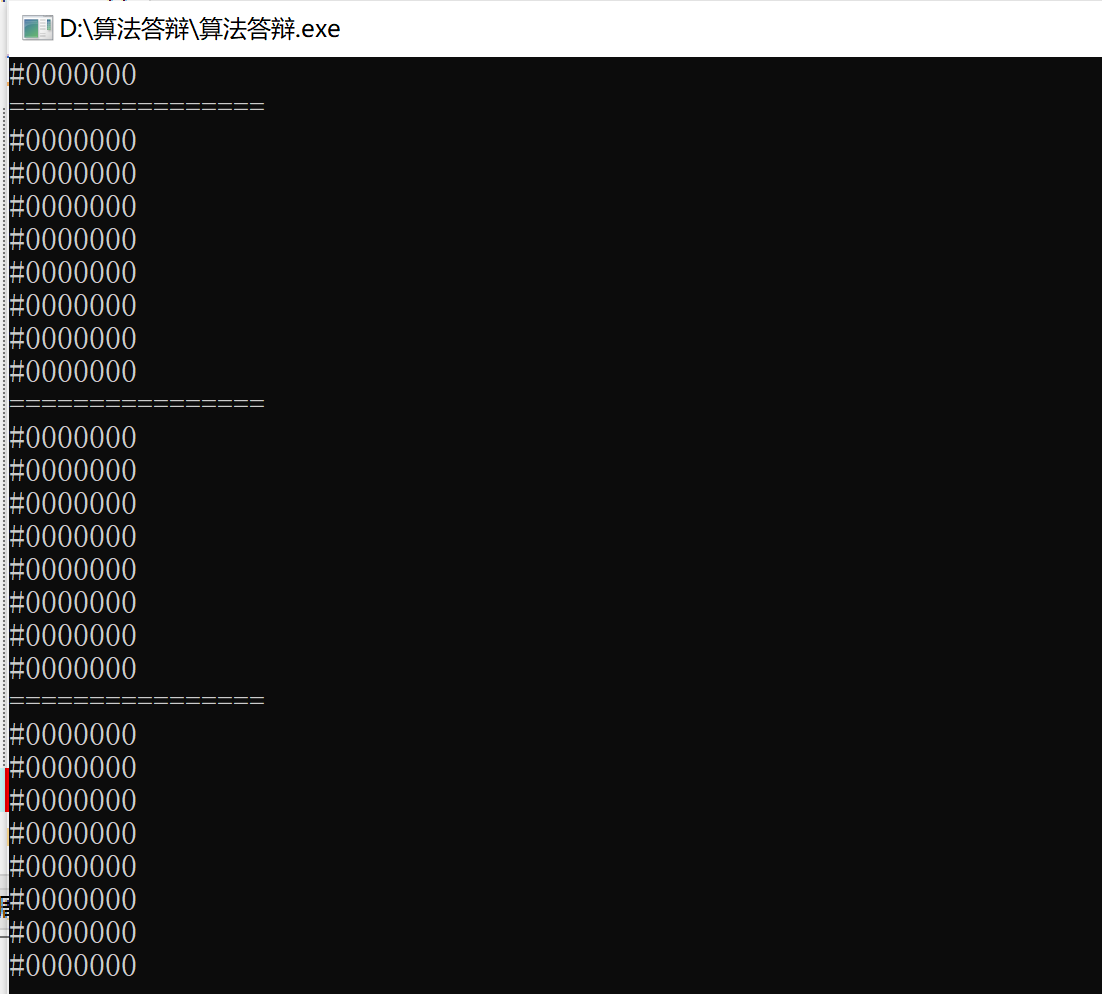图3-1

之后我们对代码进行了检查，发现对于固定的行列，没有检查是否和之前的皇后位置冲突。之后我们增添了代码对这部分进行完善。

for (int list=0; list<8; list++) {

//对于固定的行列，检查是否和之前的皇后位置冲突

if (Check(line, list)) {

Queenes[line]=list;

最终，我们成功输出了正确的结果。如图：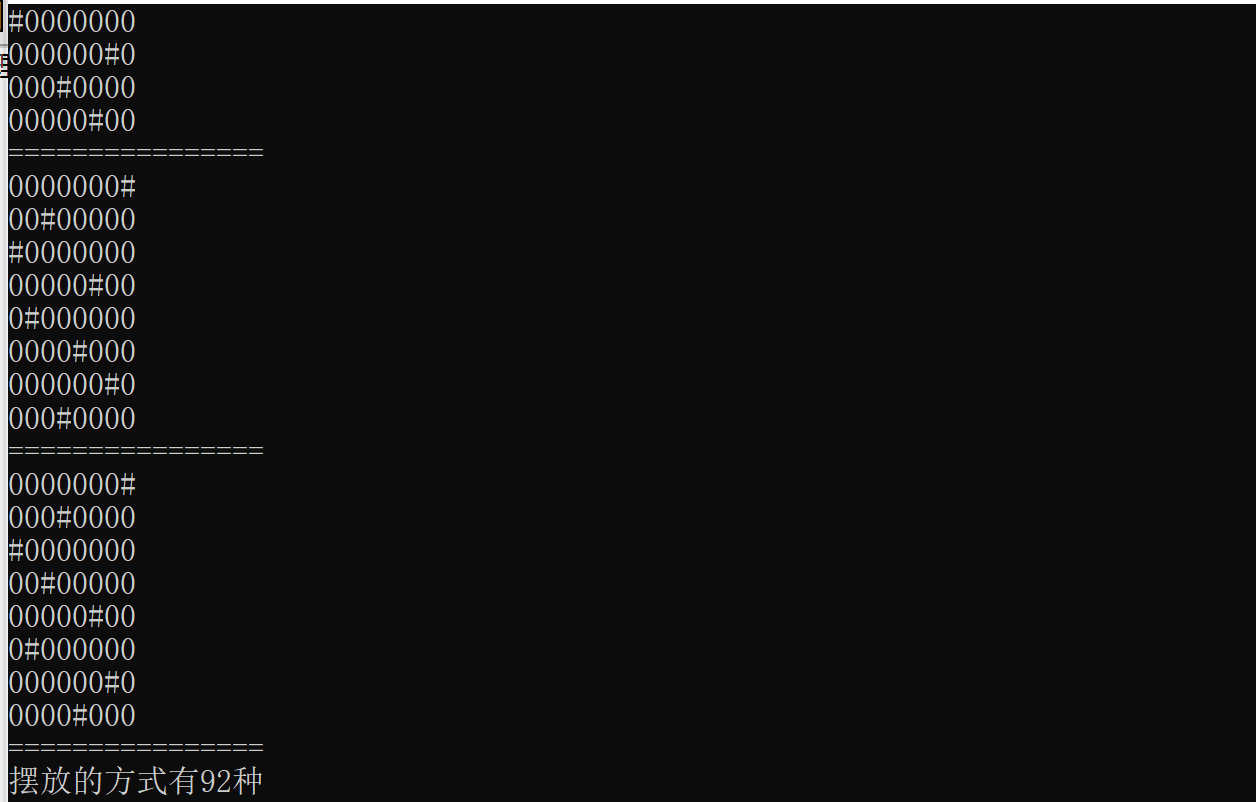图3-2

## 3.3棋盘的可视化问题

在代码编写的初始阶段，我们输出“#”为皇后，“0”为空白区域，输出结果即是由“#”和“0”构成的8×8的矩阵，并不能作为可视化的结果。如图：图3-3

可视化是将数据转换成图形或图像在屏幕上显示出来，再进行交互处理的理论、方法和技术。之后我们发现，可以用“■”代替“#”，用“□”代替“0”。输出的矩阵由“■”和“□”表示，即可表示为可视化的结果。如图：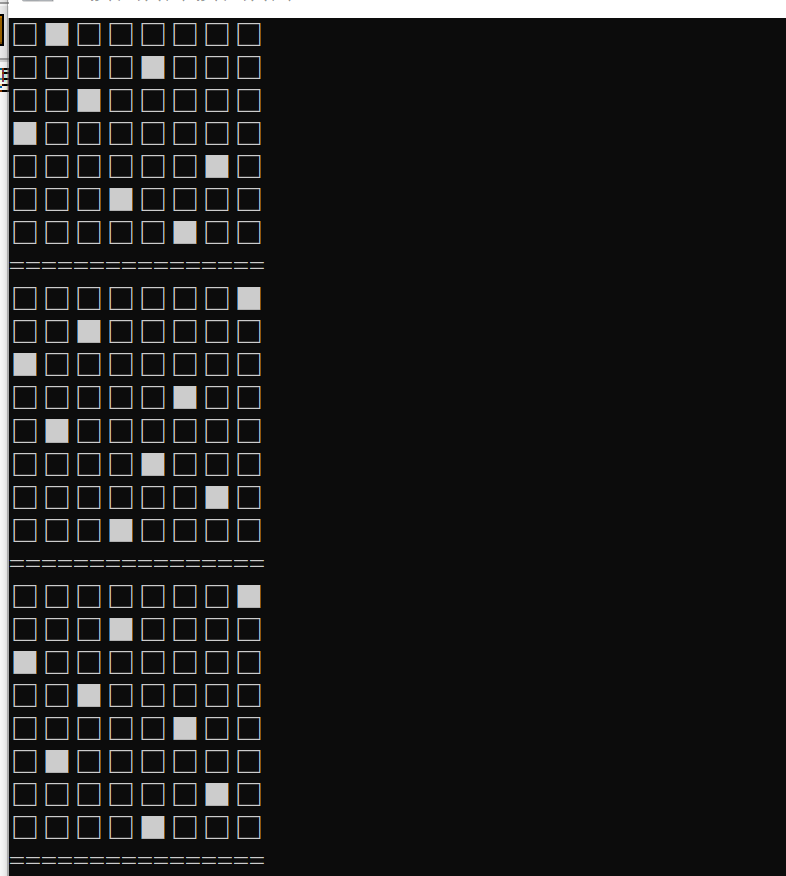图3-4

# 四、算法设计特色及关键技术

## 4.1算法设计特色

算法主要使用回溯法，又被称为“试探法”。解决问题时，每进行一步，都是抱着试试看的态度，如果发现当前选择并不是最好的，或者这么走下去肯定达不到目标，立刻做回退操作重新选择。这种走不通就回退再走的方法就是回溯法。回溯法是从初始状态出发，按照深度优先搜索的方式，根据产生子结点的条件约束，搜索问题的解。当发现当前结点不满足求解条件时，就回溯，尝试其他的路径。回溯法是一种“能进则进,进不了则换，换不了则退”的搜索方法。

## 4.2算法关键技术

### 4.2.1回溯法的实质

实际上是建立一棵“状态树”的过程,例如下图。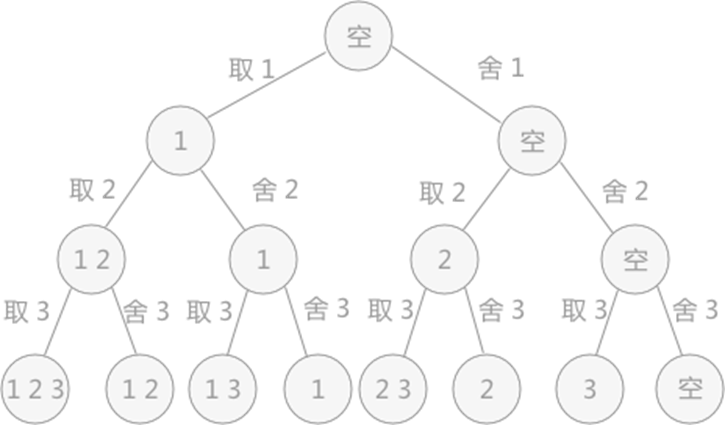图4-1

回溯法的求解过程实质上是先序遍历“状态树”的过程。树中每一个叶子结点，都有可能是问题的答案。图 中的状态树是满二叉树，得到的叶子结点全部都是问题的解。

在某些情况下，回溯法解决问题的过程中创建的状态树并不都是满二叉树，因为在试探的过程中，有时会发现此种情况下，再往下进行没有意义，所以会放弃这条死路，回溯到上一步。在树中的体现，就是在树的最后一层不是满的，即不是满二叉树，需要自己判断哪些叶子结点代表的是正确的结果。

### 4.2.2搜索过程

从根开始，以深度优先搜索的方式进行搜索。根结点是活结点，并且是当前的扩展结点。在搜索过程中，当前的扩展结点沿纵深方向移向一个新结点,判断该新结点是否满足隐约束。如果满足，则新结点成为活结点，并且成为当前的扩展结点，继续深一层的搜索;如果不满足，则换到该新结点的兄弟结点继续搜索;如果新结点没有兄弟结点，或其兄弟结点已全部搜索完毕，则扩展结点成为死结点，搜索回溯到其父结点处继续进行。搜索过程直到找到问题的根结点变成死结点为止。

### 4.2.3运行过程

（1）从棋盘的第一行开始，从第一个位置开始，依次判断当前位置是否能够放置皇后，判断的依据为：同该行之前的所有行中皇后的所在位置进行比较，如果在同一列，或者在同一条斜线上（斜线有两条，为正方形的两个对角线），都不符合要求，继续检验后序的位置。

（2）如果该行所有位置都不符合要求，则回溯到前一行，改变皇后的位置，继续试探。

如果试探到最后一行，所有皇后摆放完毕，则直接打印出 8*8 的棋盘。最后一定要记得将棋盘恢复原样，避免影响下一次摆放。

# 五、算法测试

## 5.1算法代码

#include <stdio.h>

int Queenes={0},Counts=0;

int Check(int line,int list){

//遍历该行之前的所有行

for (int index=0; index<line; index++) {

//挨个取出前面行中皇后所在位置的列坐标

int data=Queenes[index];

//如果在同一列，该位置不能放

if (list==data) {

return 0;

}

//如果当前位置的斜上方有皇后，在一条斜线上，也不行

if ((index+data)==(line+list)) {

return 0;

}

//如果当前位置的斜下方有皇后，在一条斜线上，也不行

if ((index-data)==(line-list)) {

return 0;

}

}

//如果以上情况都不是，当前位置就可以放皇后

return 1;

}

//输出语句

void print()

{

for (int line = 0; line < 8; line++)

{

int list;

for (list = 0; list < Queenes[line]; list++)

printf("□");

printf("■");

for (list = Queenes[line] + 1; list < 8; list++){

printf("□");

}

printf("\n");

}

printf("================\n");

}

void eight_queen(int line){

//在数组中为0-7列

for (int list=0; list<8; list++) {

//对于固定的行列，检查是否和之前的皇后位置冲突

if (Check(line, list)) {

//不冲突，以行为下标的数组位置记录列数

Queenes[line]=list;

//如果最后一样也不冲突，证明为一个正确的摆法

if (line==7) {

//统计摆法的Counts加1

Counts++;

//输出这个摆法

print();

//每次成功，都要将数组重归为0

Queenes[line]=0;

return;

}

//继续判断下一样皇后的摆法，递归

eight_queen(line+1);

//不管成功失败，该位置都要重新归0，以便重复使用。

Queenes[line]=0;

}

}

}

int main() {

//调用回溯函数，参数0表示从棋盘的第一行开始判断

eight_queen(0);

printf("摆放的方式有%d种",Counts);

return 0;

}

## 5.2运行结果

八个皇后在8x8棋盘上共有4,426,165,368种摆放方法，但只有92个互不相同的解。如果将旋转和对称的解归为一种的话，则一共有12个独立解，具体如下：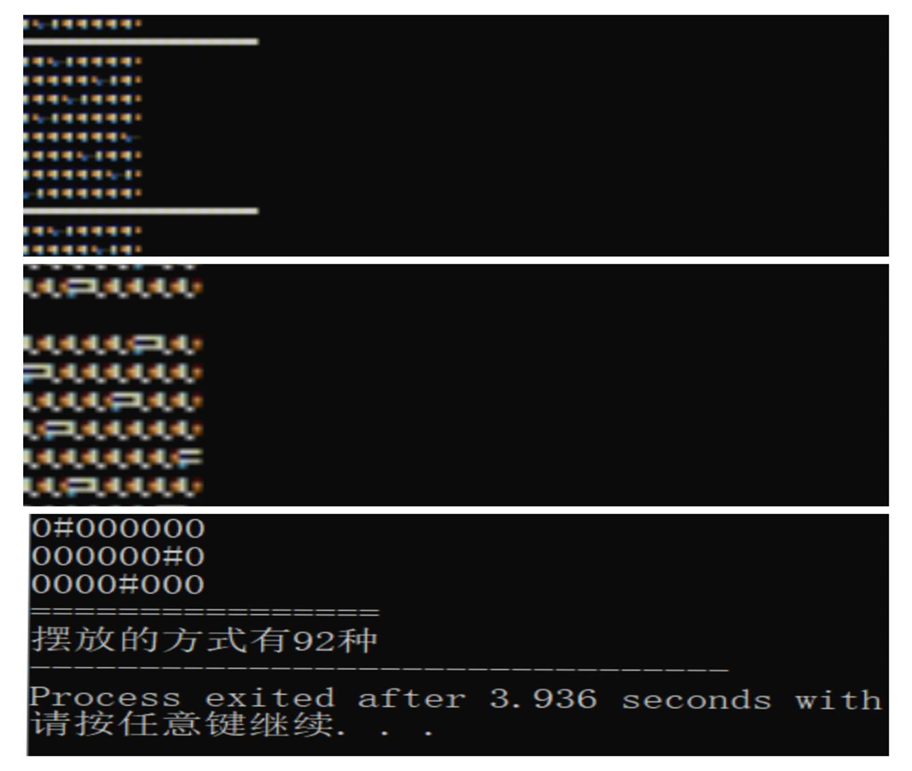图5-1

以上为全部解。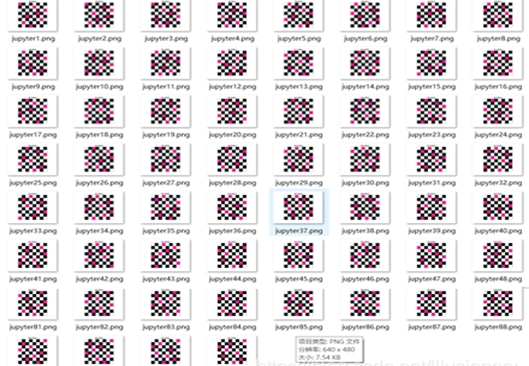图5-2

上图为所有可能的八皇后位置。

## 5.3算法性能测试

程序运行耗时3.936s。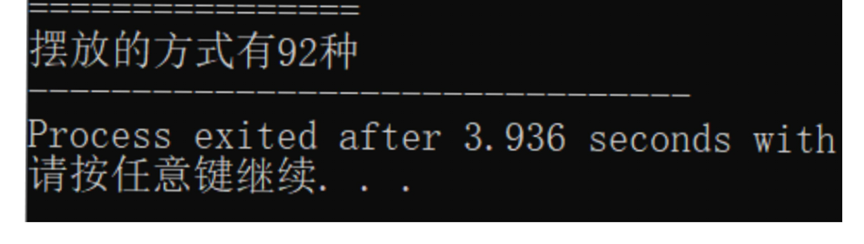图5-3

# 六、结论

在某些情况下，回溯法解决问题的过程中创建的状态树并不都是满二叉树，因为在试探的过程中，有时会发现此种情况下，再往下进行没有意义，所以会放弃这条死路，回溯到上一步。在树中的体现，就是在树的最后一层不是满的，即不是满二叉树，需要自己判断哪些叶子结点代表的是正确的结果。

回溯法可以系统的搜索一个问题的所有解或任意解，回溯法在问题的解空间树中，按深度优先策略，从根节点出发搜索解空间树。算法搜索至解空间树的任一结点时，先判断该结点是否包含问题的解，如果肯定不包含，则跳过对以该结点为根的子树的搜索，逐层向其祖先结点回溯。否则，进入该子树，继续按深度优先策略搜索。它适合于解组合数较大的问题。

展开全文• 回溯法回溯法回溯法回溯法回溯法回溯法回溯法回溯法回溯法回溯法回溯法回溯法回溯法
• 主要介绍了C语言基于回溯算法解决八皇后问题的方法,简单描述了八皇后问题,并结合实例形式分析了C语言使用回溯算法解决八皇后问题的相关操作技巧,需要的朋友可以参考下
• 八皇后问题是一个以国际象棋为背景的问题：如何能够在 8×8 的国际象棋棋 盘上放置八个皇后，使得任何一个皇后都无法直接吃掉其他的皇后。为了达 到此目的，任两个皇后都不能处于同一条横行、纵行或斜线上。 实验...

## 实验内容

八皇后问题是一个以国际象棋为背景的问题：如何能够在 8×8 的国际象棋棋 盘上放置八个皇后，使得任何一个皇后都无法直接吃掉其他的皇后。为了达 到此目的，任两个皇后都不能处于同一条横行、纵行或斜线上。

## 实验原理

按照 DFS 算法的策略，从跟结点出发搜索解空间树。首先根结点成为活结点， 同时也成为当前的扩展结点。在当前的扩展结点处，搜索向纵深方向移至一个新结点。这个新结点就成为新的活结点，并成为当前扩展结点。如果在当前的扩展结点处不能再向纵深方向移动，则当前扩展结点就成了死结点。此时应往回移动（回溯）至最近的一个活结点处，并使这个活结点成为当前的扩展结点。 回溯法以这种方式递归地在解空间中搜索，直到找到所要求的解或解空间中已无活结点为止。

## 实验步骤

数组法：（这里采用该方法实现）
① 根据 8 皇后问题，可以把其设想为一个数组；
② 根据 8 皇后的规则，可以设想为数组上同一直线，横线，斜线的数字都 不 能相同，由此可以得出判断条件；
③ 根据判断条件之后，建立回溯点，即可解决问题。
堆栈法：
① 检索当前行是否可以放置一个皇后；
② 利用检索过程，通过递归的方式，来确定每个皇后的位置———回溯的思想。

## 代码实现

import time

def show(queen):
for i in range(0, 8):
print("(" + str(i + 1) + ", " + str(queen[i] + 1) + ")", end = " ")
print("\n")

def isNotConfict(queen, row):   #判断是否冲突
for i in range(0, row):
if queen[i] == queen[row] or abs(i - row) == abs(queen[i] - queen[row]):
return False
return True

sum = 0                         #记录解法总数

def put_queen(queen, row):      #回溯法放置皇后
if row == len(queen):       #如果到达最后一行，那么肯定得到一种新解法
show(queen)             #输出皇后位置
global sum
sum += 1                #更新解法总数
return sum
for column in range(0, len(queen)):#循环判断每列是否可以放置皇后
queen[row] = column
if isNotConfict(queen, row):
put_queen(queen, row + 1)

def main():
queen = [None] * 8
start = time.time()
put_queen(queen, 0)
end = time.time()
print("共耗时:\n" + str(end - start) + " s\n")
print("一共有" + str(sum) + "种解法")

if __name__ == '__main__':
main()


## 时间复杂度分析

因为回溯法遇到当前解不可行时就会回溯到上一结点，因此时间复杂度应该没有达到O(n!)，看了很多大佬都说是O(n3)，但是都没有求解过程，因此这里想请教各位访客如何求解该算法的时间复杂度。

## 运行结果

(1, 1) (2, 5) (3, 8) (4, 6) (5, 3) (6, 7) (7, 2) (8, 4)

(1, 1) (2, 6) (3, 8) (4, 3) (5, 7) (6, 4) (7, 2) (8, 5)

(1, 1) (2, 7) (3, 4) (4, 6) (5, 8) (6, 2) (7, 5) (8, 3)

(1, 1) (2, 7) (3, 5) (4, 8) (5, 2) (6, 4) (7, 6) (8, 3)

(1, 2) (2, 4) (3, 6) (4, 8) (5, 3) (6, 1) (7, 7) (8, 5)

(1, 2) (2, 5) (3, 7) (4, 1) (5, 3) (6, 8) (7, 6) (8, 4)

(1, 2) (2, 5) (3, 7) (4, 4) (5, 1) (6, 8) (7, 6) (8, 3)

(1, 2) (2, 6) (3, 1) (4, 7) (5, 4) (6, 8) (7, 3) (8, 5)

(1, 2) (2, 6) (3, 8) (4, 3) (5, 1) (6, 4) (7, 7) (8, 5)

(1, 2) (2, 7) (3, 3) (4, 6) (5, 8) (6, 5) (7, 1) (8, 4)

(1, 2) (2, 7) (3, 5) (4, 8) (5, 1) (6, 4) (7, 6) (8, 3)

(1, 2) (2, 8) (3, 6) (4, 1) (5, 3) (6, 5) (7, 7) (8, 4)

(1, 3) (2, 1) (3, 7) (4, 5) (5, 8) (6, 2) (7, 4) (8, 6)

(1, 3) (2, 5) (3, 2) (4, 8) (5, 1) (6, 7) (7, 4) (8, 6)

(1, 3) (2, 5) (3, 2) (4, 8) (5, 6) (6, 4) (7, 7) (8, 1)

(1, 3) (2, 5) (3, 7) (4, 1) (5, 4) (6, 2) (7, 8) (8, 6)

(1, 3) (2, 5) (3, 8) (4, 4) (5, 1) (6, 7) (7, 2) (8, 6)

(1, 3) (2, 6) (3, 2) (4, 5) (5, 8) (6, 1) (7, 7) (8, 4)

(1, 3) (2, 6) (3, 2) (4, 7) (5, 1) (6, 4) (7, 8) (8, 5)

(1, 3) (2, 6) (3, 2) (4, 7) (5, 5) (6, 1) (7, 8) (8, 4)

(1, 3) (2, 6) (3, 4) (4, 1) (5, 8) (6, 5) (7, 7) (8, 2)

(1, 3) (2, 6) (3, 4) (4, 2) (5, 8) (6, 5) (7, 7) (8, 1)

(1, 3) (2, 6) (3, 8) (4, 1) (5, 4) (6, 7) (7, 5) (8, 2)

(1, 3) (2, 6) (3, 8) (4, 1) (5, 5) (6, 7) (7, 2) (8, 4)

(1, 3) (2, 6) (3, 8) (4, 2) (5, 4) (6, 1) (7, 7) (8, 5)

(1, 3) (2, 7) (3, 2) (4, 8) (5, 5) (6, 1) (7, 4) (8, 6)

(1, 3) (2, 7) (3, 2) (4, 8) (5, 6) (6, 4) (7, 1) (8, 5)

(1, 3) (2, 8) (3, 4) (4, 7) (5, 1) (6, 6) (7, 2) (8, 5)

(1, 4) (2, 1) (3, 5) (4, 8) (5, 2) (6, 7) (7, 3) (8, 6)

(1, 4) (2, 1) (3, 5) (4, 8) (5, 6) (6, 3) (7, 7) (8, 2)

(1, 4) (2, 2) (3, 5) (4, 8) (5, 6) (6, 1) (7, 3) (8, 7)

(1, 4) (2, 2) (3, 7) (4, 3) (5, 6) (6, 8) (7, 1) (8, 5)

(1, 4) (2, 2) (3, 7) (4, 3) (5, 6) (6, 8) (7, 5) (8, 1)

(1, 4) (2, 2) (3, 7) (4, 5) (5, 1) (6, 8) (7, 6) (8, 3)

(1, 4) (2, 2) (3, 8) (4, 5) (5, 7) (6, 1) (7, 3) (8, 6)

(1, 4) (2, 2) (3, 8) (4, 6) (5, 1) (6, 3) (7, 5) (8, 7)

(1, 4) (2, 6) (3, 1) (4, 5) (5, 2) (6, 8) (7, 3) (8, 7)

(1, 4) (2, 6) (3, 8) (4, 2) (5, 7) (6, 1) (7, 3) (8, 5)

(1, 4) (2, 6) (3, 8) (4, 3) (5, 1) (6, 7) (7, 5) (8, 2)

(1, 4) (2, 7) (3, 1) (4, 8) (5, 5) (6, 2) (7, 6) (8, 3)

(1, 4) (2, 7) (3, 3) (4, 8) (5, 2) (6, 5) (7, 1) (8, 6)

(1, 4) (2, 7) (3, 5) (4, 2) (5, 6) (6, 1) (7, 3) (8, 8)

(1, 4) (2, 7) (3, 5) (4, 3) (5, 1) (6, 6) (7, 8) (8, 2)

(1, 4) (2, 8) (3, 1) (4, 3) (5, 6) (6, 2) (7, 7) (8, 5)

(1, 4) (2, 8) (3, 1) (4, 5) (5, 7) (6, 2) (7, 6) (8, 3)

(1, 4) (2, 8) (3, 5) (4, 3) (5, 1) (6, 7) (7, 2) (8, 6)

(1, 5) (2, 1) (3, 4) (4, 6) (5, 8) (6, 2) (7, 7) (8, 3)

(1, 5) (2, 1) (3, 8) (4, 4) (5, 2) (6, 7) (7, 3) (8, 6)

(1, 5) (2, 1) (3, 8) (4, 6) (5, 3) (6, 7) (7, 2) (8, 4)

(1, 5) (2, 2) (3, 4) (4, 6) (5, 8) (6, 3) (7, 1) (8, 7)

(1, 5) (2, 2) (3, 4) (4, 7) (5, 3) (6, 8) (7, 6) (8, 1)

(1, 5) (2, 2) (3, 6) (4, 1) (5, 7) (6, 4) (7, 8) (8, 3)

(1, 5) (2, 2) (3, 8) (4, 1) (5, 4) (6, 7) (7, 3) (8, 6)

(1, 5) (2, 3) (3, 1) (4, 6) (5, 8) (6, 2) (7, 4) (8, 7)

(1, 5) (2, 3) (3, 1) (4, 7) (5, 2) (6, 8) (7, 6) (8, 4)

(1, 5) (2, 3) (3, 8) (4, 4) (5, 7) (6, 1) (7, 6) (8, 2)

(1, 5) (2, 7) (3, 1) (4, 3) (5, 8) (6, 6) (7, 4) (8, 2)

(1, 5) (2, 7) (3, 1) (4, 4) (5, 2) (6, 8) (7, 6) (8, 3)

(1, 5) (2, 7) (3, 2) (4, 4) (5, 8) (6, 1) (7, 3) (8, 6)

(1, 5) (2, 7) (3, 2) (4, 6) (5, 3) (6, 1) (7, 4) (8, 8)

(1, 5) (2, 7) (3, 2) (4, 6) (5, 3) (6, 1) (7, 8) (8, 4)

(1, 5) (2, 7) (3, 4) (4, 1) (5, 3) (6, 8) (7, 6) (8, 2)

(1, 5) (2, 8) (3, 4) (4, 1) (5, 3) (6, 6) (7, 2) (8, 7)

(1, 5) (2, 8) (3, 4) (4, 1) (5, 7) (6, 2) (7, 6) (8, 3)

(1, 6) (2, 1) (3, 5) (4, 2) (5, 8) (6, 3) (7, 7) (8, 4)

(1, 6) (2, 2) (3, 7) (4, 1) (5, 3) (6, 5) (7, 8) (8, 4)

(1, 6) (2, 2) (3, 7) (4, 1) (5, 4) (6, 8) (7, 5) (8, 3)

(1, 6) (2, 3) (3, 1) (4, 7) (5, 5) (6, 8) (7, 2) (8, 4)

(1, 6) (2, 3) (3, 1) (4, 8) (5, 4) (6, 2) (7, 7) (8, 5)

(1, 6) (2, 3) (3, 1) (4, 8) (5, 5) (6, 2) (7, 4) (8, 7)

(1, 6) (2, 3) (3, 5) (4, 7) (5, 1) (6, 4) (7, 2) (8, 8)

(1, 6) (2, 3) (3, 5) (4, 8) (5, 1) (6, 4) (7, 2) (8, 7)

(1, 6) (2, 3) (3, 7) (4, 2) (5, 4) (6, 8) (7, 1) (8, 5)

(1, 6) (2, 3) (3, 7) (4, 2) (5, 8) (6, 5) (7, 1) (8, 4)

(1, 6) (2, 3) (3, 7) (4, 4) (5, 1) (6, 8) (7, 2) (8, 5)

(1, 6) (2, 4) (3, 1) (4, 5) (5, 8) (6, 2) (7, 7) (8, 3)

(1, 6) (2, 4) (3, 2) (4, 8) (5, 5) (6, 7) (7, 1) (8, 3)

(1, 6) (2, 4) (3, 7) (4, 1) (5, 3) (6, 5) (7, 2) (8, 8)

(1, 6) (2, 4) (3, 7) (4, 1) (5, 8) (6, 2) (7, 5) (8, 3)

(1, 6) (2, 8) (3, 2) (4, 4) (5, 1) (6, 7) (7, 5) (8, 3)

(1, 7) (2, 1) (3, 3) (4, 8) (5, 6) (6, 4) (7, 2) (8, 5)

(1, 7) (2, 2) (3, 4) (4, 1) (5, 8) (6, 5) (7, 3) (8, 6)

(1, 7) (2, 2) (3, 6) (4, 3) (5, 1) (6, 4) (7, 8) (8, 5)

(1, 7) (2, 3) (3, 1) (4, 6) (5, 8) (6, 5) (7, 2) (8, 4)

(1, 7) (2, 3) (3, 8) (4, 2) (5, 5) (6, 1) (7, 6) (8, 4)

(1, 7) (2, 4) (3, 2) (4, 5) (5, 8) (6, 1) (7, 3) (8, 6)

(1, 7) (2, 4) (3, 2) (4, 8) (5, 6) (6, 1) (7, 3) (8, 5)

(1, 7) (2, 5) (3, 3) (4, 1) (5, 6) (6, 8) (7, 2) (8, 4)

(1, 8) (2, 2) (3, 4) (4, 1) (5, 7) (6, 5) (7, 3) (8, 6)

(1, 8) (2, 2) (3, 5) (4, 3) (5, 1) (6, 7) (7, 4) (8, 6)

(1, 8) (2, 3) (3, 1) (4, 6) (5, 2) (6, 5) (7, 7) (8, 4)

(1, 8) (2, 4) (3, 1) (4, 3) (5, 6) (6, 2) (7, 7) (8, 5)

共耗时:
0.05162310600280762 s

一共有92种解法

展开全文算法 python
• #利用回溯法解决八皇后问题 这个地方的回溯不好理解，强调一下 为什么回溯 假如现在放的是第六个皇后，其实是在check（第5个皇后）中调用了check（第6个），从第一列位置开始尝试放置皇后，直到最后一个位置都在冲突...数据结构 c++ 算法
• 本文主要是给出通过Python回溯法计算八皇后问题，除了在打印结果部分有点麻烦外，计算过程相对简单。 """ 八皇后问题是指如何在一个8X8的棋盘中放置八个皇后，并令他们相互攻击不到对方。也即每一行、每一列和每条...python 数据结构
• 一、回溯法回溯法是一个既带有系统性又带有跳跃性的的搜索算法。它在包含问题的所有解的解空间树中，按照深度优先的策略，从根结点出发搜索解...回溯法在用来求问题的所有解时，要回溯到根，且根结点的所有子树都...
• 八皇后问题 递归（回溯法求解），很简洁，欢迎下载交流。
• 继上次学完函数之后，这次来通过一个实例来加深印象，下面会对其实现过程进行一一剖新，先看一下什么叫“八皇后问题” 具体的算法可以分解为： 像上图中第五行就已经出现了死胡同，这时应该退到第四行，重新...算法 c语言
• 小弟申请ID不久，资源分用的很快，自己写点程序借此赚分以备使用。初出茅庐，学识尚浅。请各路朋友多多指点，不胜感激。
• 最近感觉有些迷惘不知道做什么，于是翻了下算法，看到了八皇后问题，于是研究了一下。Java代码如下public class Queen8 { static int sum=0; final static int n=8; static int[] x=new int; public static...java
• 八皇后问题（英文：Eight queens），是由国际西洋棋棋手马克斯·贝瑟尔于1848年提出的问题，是回溯算法的典型案例。 问题表述为：在8×8格的国际象棋上摆放8个皇后，使其不能互相攻击，即任意两个皇后都不能处于...
• 主要介绍了C语言八皇后问题解决方法,简单描述了八皇后问题并结合实例形式分析了C语言基于暴力法与回溯法解决八皇后的具体操作技巧,需要的朋友可以参考下
• # include using namespace std ; int row_col [ 8 ] ; int count = 0 ; bool isOk ( int row , int col ) { int ..."八皇后问题一共有" count "种可能" ; }算法
• 八皇后问题是典型的回溯法解决的问题，以这个问题为例介绍回溯法。 首先利用回溯算法，先给第一个皇后安排位置，如下图所示，安排在（1,1）然后给第二个皇后安排位置，可知（2,1），（2,2）都会产生冲突，因此可以...java
• 要求用回溯法求解 8-皇后问题，使放置在 8*8 棋盘上的 8 个皇后彼此不受攻击，即：任 何两个皇后都不在同一行、同一列或同一斜线上。请输出 8 皇后问题的所有可行解。 问题分析 在解决八皇后问题时，如果采用穷举法...算法 c++
• 八皇后问题，是一个古老而著名的问题，是回溯算法的典型案例。该问题是国际西洋棋棋手马克斯·贝瑟尔于1848年提出：在8×8格的国际象棋上摆放八个皇后，使其不能互相攻击，即任意两个皇后都不能处于同一行、同一列或...
• 问题描述：要求在8x8的国际象棋棋盘上放入皇后，使得任意两个皇后不能处于同一列，同一行，同一条斜线上。 算法思路： 1） 判断皇后之间有没有冲突，要引入四个量–row（行号，也对应于皇后的编号），column[ ]...C语言
• 1,什么是回溯? 回溯算法实际上一个类似枚举的搜索尝试过程,主要是在搜索尝试过程中寻找问题的解,当发现已经不满足求解条件时,就"回溯"返回,尝试别的路径。(走不通就退回去再走的技术) ...八皇后问题就是:在 8× 8算法 数据结构 递归算法
• //回溯法求解八皇后问题 //Linruier #include&lt;iostream&gt; #include&lt;algorithm&gt; #include&lt;cmath&gt; using namespace std; bool ok(int a[],int num) { if(num==0)return true...
• 回溯法，又被称为“试探法”。解决问题时，每进行一步，都是抱着试试看的态度，如果发现当前选择并不是最好的，或者这么走下去肯定达不到目标，立刻做回退操作... 回溯法问题本身出发，寻找可能实现的所有情况......